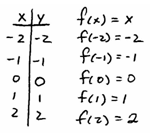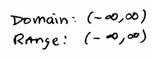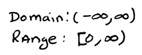## Algebra

Showing posts with label basic functions. Show all posts
Showing posts with label basic functions. Show all posts

### Graphing Basic Functions

The graphs of the following basic functions can be determined by plotting points. That is, choose some x-values and then substitute them in to find the corresponding y-values. The more points you plot the better the picture. [ Free Printable Graph Paper ]

Graph f(x) = x and state the domain and range.Squaring Function: [ https://www.desmos.com/calculator/rslqgtrovn ]
Graph f(x) = x^2 and state the domain and range.Graph f(x) = x^3 and state the domain and range.Absolute Value Function: https://www.desmos.com/calculator/au6i2qvsyf ]
Graph f(x) = | | and state the domain and range.Graph f(x) = sqrt(x) and state the domain and range.Graph f(x) = 1/x and state the domain and range.